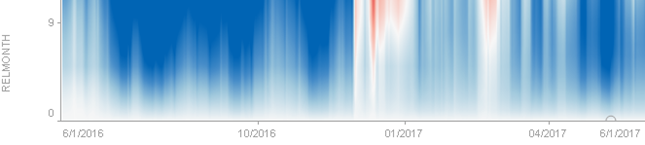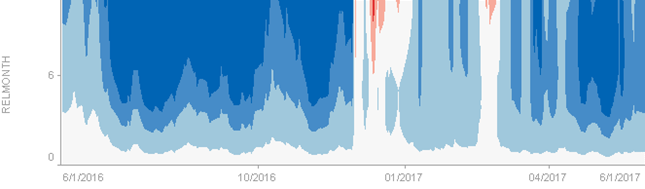Altair® Panopticon

# Time Series Surface Plot

Time Series Surface Plots are used to identify trends and outliers within Time Series surfaces, typically forward curves across time.

The Surface is made up of a series of points where each point has:

• Time Position

• Y Position

• Color (which represents the Z axis)

The color scale can be continuous or stepped to show a surface gradient.

The below figures show an Oil Futures forward curve, with prices relative to the front month.Figure 1. A time series surface plot with continuous colorsFigure 2. A time series surface plot with stepped colors